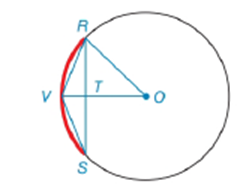Chapter 6.3, Problem 3E### Elementary Geometry for College St...

6th Edition
Daniel C. Alexander + 1 other
ISBN: 9781285195698

#### Solutions

Chapter
Section### Elementary Geometry for College St...

6th Edition
Daniel C. Alexander + 1 other
ISBN: 9781285195698
Textbook Problem
132 views

# Note: Exercises preceded by an asterisk are of a more challenging nature.Given: O V ¯ ⊥ R S ¯     i n     ⊙ O O V = 9     a n d     O T = 6 Find: R STo determine

To find:

To find RS.

Explanation

Given that OV¯RS¯ in O,OV=9 and OT=6

Consider the following figure,

Given that OV¯RS¯ in O then ΔORT is right triangle.

Pythagorean theorem:

In a right angled triangle then the square of the hypotenuse is equal to the sum of the squares of the other two sides.

Also OR=OV since radius are same for all points on a circle.

Consider the ΔORT to get the following,

OR2=OT2+TR2

Substitute the value of OV=OR=9 and OT=6 to get the following,

### Still sussing out bartleby?

Check out a sample textbook solution.

See a sample solution

#### The Solution to Your Study Problems

Bartleby provides explanations to thousands of textbook problems written by our experts, many with advanced degrees!

Get Started

#### Find more solutions based on key concepts